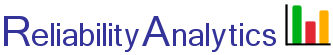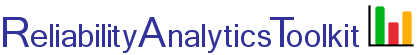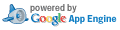# Field MTBF Calculator

This tool computes the lower one-sided MTBF at a given confidence limit based on the number of unit-hours accumulated and the total number of field failures. The calculation assumes that units have a constant failure rate and fail in accordance with the exponential distribution. For purposes of this calculation it is assumed that the "field test" is time truncated, thus making use of equation 2 shown for this tool. One common use of the tool is to estimate the current MTBF for a population of fielded units when no failures have occurred, which is typically calculated at a 60% confidence level.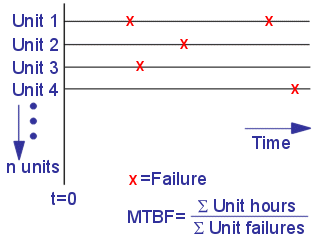## Calculation Inputs:

1. Select method:
Method 1
Method 2
2. Cumulative unit-hours (t, hours):
2a. Number of units in the field (n):
2b. Average time each unit has been been fielded :
2c. Operating duty cycle (dc, 0-1.0):
3. Cumulative field failures (r):
4. Confidence level: Table:
5. Decimal places:

## Featured Reference: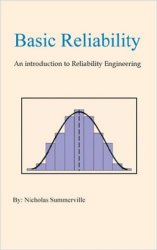Basic Reliability: An introduction to Reliability Engineering

Toolkit Home